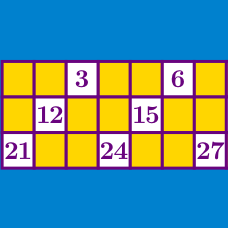Number Theory

# Application of Divisibility Rules

Find the digit $N$ such that the three digit integer $\overline{ 5 7 N}$ is a multiple of 9.

How many digits $N$ are there such that the six-digit integer $\overline{1 2 3 3 N 2}$ is a multiple of 8?

Find the digit $N$ such that the five-digit number $\overline{N878N}$ is divisible by 9.

How many three-digit numbers are not divisible by $5,$ have digits that sum to $15,$ and have the first digit equal to the third digit?

How many 5 digit positive integers are multiples of 11, and have digits which sum to 43?

×